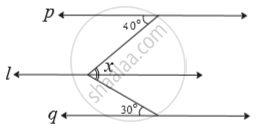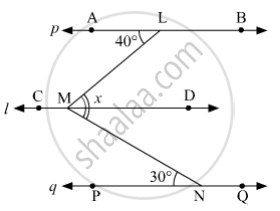SSC (English Medium) Class 8Maharashtra State Board
Share

# In the Adjoining Figure, Line P || Line L || Line Q. Find ∠X with the Help of the Measures Given in the Figure. - SSC (English Medium) Class 8 - Mathematics

ConceptProperties of Angles Formed by Two Parallel Lines and a Transversal

#### Question

In the adjoining figure, line p || line l || line q.  Find ∠x with the help of the measures given in the figure.#### SolutionLet us mark the points A, L and B on p; C, M and D on l; P, N and Q on q.
Since, AB||CD and LM is a transversal intersecting AB at L and CD at M, then
m∠LMD = ​m∠ALM    (Alternate interior angles)
⇒ m∠LMD = 40°
Since, CD||PQ and MN is a transversal intersecting CD at M and PQ at N, then
m∠DMN = ​m∠PNM    (Alternate interior angles)
⇒ m∠DMN = 30°
Now, m∠LMD + m∠DMN = 40° + 30°
⇒ m∠LMN = 70°
⇒ x = 70°

Is there an error in this question or solution?

#### APPEARS IN

Balbharati Solution for Balbharati Class 8 Mathematics (2019 to Current)
Chapter 2: Parallel lines and transversals
Practice Set 2.2 | Q: 5 | Page no. 12
Solution In the Adjoining Figure, Line P || Line L || Line Q. Find ∠X with the Help of the Measures Given in the Figure. Concept: Properties of Angles Formed by Two Parallel Lines and a Transversal.
S